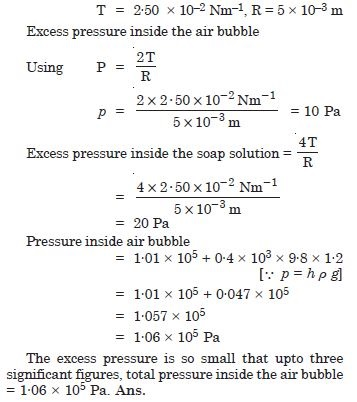# NCERT Solutions for Class 11 Physics Chapter 10 - Mechanical Properties of Fluids

##### Question 1:

Explain why
The blood pressure in human is greater at feet than at brain.

The height of the blood column in the human body is more for feet than for brain. So the blood exerts more pressure at the feet than at the brain.

##### Question 2:

Explain why
Atmospheric pressure at a height of about 6 km decreases nearly to half its value at the sea level though the height of the atmosphere is more than 1000 km.

Because density falls nearly half its value of surface and this height decreases rather very slowly.

##### Question 3:

Explain why
Hydrostatic pressure is a scalar quantity even though pressure is force divided by area.

The direction of force acting on a fluid in m is always specified, the force is completely described by its magnitude and hence the hydrostatic pressure can be completely determined by the magnitude of force acting per unit area and so hydrostatic pressure can be taken to be a scalar quantity.

##### Question 4:

Explain why
The angle of contact of mercury with glass is obtuse, while that of water with glass is acute.

Water wets the surface of glass and rises along the surface. Hence its meniscus is concave upward and therefore the angle of contact is acute. While mercury does not wet the surface of the glass, mercury is depressed. Its meniscus concave downward and the angle of contact is obtuse (140$°$).

##### Question 5:

Explain why
Water on a clean glass surface tends to spread out while mercury on the same surface tends to form drops. (Put differently, water wets the glass while mercury does not.)

Water wets the walls of glass surface because water sticks with glass because force of adhesion between glass and water molecule is greater than force of cohesion between the water molecules. The mercury does not stick with the glass because force of cohesion between the mercury molecules is greater than the force of adhesion between the mercury and glass.

##### Question 6:

Explain why
The surface tension is independent of area of surface.

The free surface of water behaves as a stretched membrane due to its surface tension. Surface tension is measured as force per unit length of imaginary line drawn on liquid surface.

##### Question 7:

Explain why
Detergents should have small angle of contact.

Because surface tension decreases.

##### Question 8:

Explain why
A drop of liquid under no external forces is always spherical in shape

The surface of liquid drop tries to shrink in area and a sphere has a minimum surface area for a given volume. Hence liquid drop attains the shape of a sphere under no external forces.

##### Question 9:

Fill in the blanks using the words from list appended with each statement :
Surface tension of liquids generally ........ with temperature (increases/decreases).

decreases.

##### Question 10:

Fill in the blanks using the words from list appended with each statement :
Viscosity of gases ................. with temperature, whereas viscosity of liquids ............ with temperature (increases/decreases).

viscosity of the gases increases, whereas viscosity of liquids decreases with temperature.

##### Question 11:

Fill in the blanks using the words from list appended with each statement :
For solids with elastic modulus of rigidity, the shearing force is proportional to ........... while for liquids it is proportional to ........... (shear strain/rate of shear strain).

shear strain, rate of shear strain.

##### Question 12:

Fill in the blanks using the words from list appended with each statement :
For a fluid in steady flow, the increase in flow speed at a constriction follows from .............., while the decrease of pressure there follows from ............ (conservation of mass/Bernoulli’s equation).

conservation of mass, Bernoulli’s equation.

##### Question 13:

Fill in the blanks using the words from list appended with each statement :
For the model of a plane in a wind tunnel, turbulence occurs at a ................ speed than the critical speed for turbulence for an actual plane (greater/smaller).

greater.

##### Question 14:

Explain why
To keep a piece of paper horizontal, you should blow over not under it.

When a piece of paper is blown over it, according to Bernoulli’s principle the pressure over it decreases than that of the pressure on lower surface, therefore, it remains horizontal.

##### Question 15:

Explain why
When we try to close a water tip with our fingers, fast jets of water rush through the openings between our finger.

Area of openings decreases thereby increasing the velocity.

##### Question 16:

Explain why
The size of needle of a syringe controls flow rate better than the thumb pressure exerted by a doctor while administering an injection.

According to the equation of continuity, decrease in area in the part of syringe increases the velocity of liquid.

##### Question 17:

Explain why
A fluid flowing out of a small hole in a vessel results a backward thrust on the vessel.

Since area of hole is small, therefore, velocity of liquid rushing out is large which result in backward thrust.

##### Question 18:

Explain why
A spinning cricket ball in air does not follow a parabolic trajectory.

Spinning of a ball. When a spherical ball is rotated about an axis perpendicular to the plane of paper, the surrounding air is set into motion in streamlines of concentric circles in a plane parallel to the plane of paper and the direction of rotation of the ball [Fig. 10.1(a)]. The more rough the surface of the sphere, the thicker is the layer of air set in motion.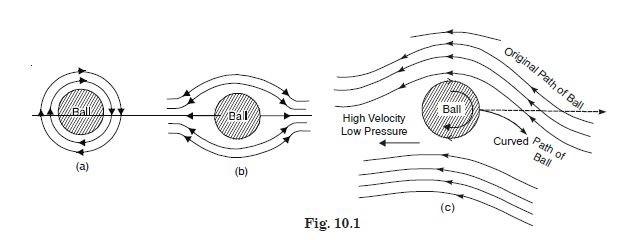When the ball is given only translatory (linear) motion forward, the air in front is pushed aside. It flows backward on the sides and towards its rear end [Fig. 10.1(b)]. However, if the ball possesses translational as well as rotatory motion, as in case of a spinning ball, [Fig. 10.1(c)] then the two streams on the upper side are in opposite directions hence the resultant velocity of air is decreased and pressure increased. On its lower side the two sets of streamlines are in the same direction and help each other. Thus, the velocity of air is increased and pressure decreased. Hence the ball experiences a net lateral force in downward direction and it is for this reason that the ball describes a curved path, which is convex on the side of greater pressure. This is called Magnus effect and is exhibited by a tennis or golf ball when given spin.

##### Question 19:

A 50 kg girl wearing high heel shoes balances on a single heel. The heel is circular with a diameter 1.0 cm. What is the pressure exerted by the heel on the horizontal floor ?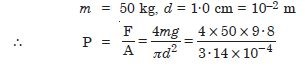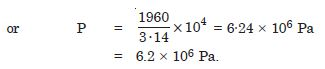##### Question 20:

Torricelli’s barometer used mercury. Pascal duplicated it using French wine of density 984 kg m–3. Determine the height of wine column for normal atmospheric pressure.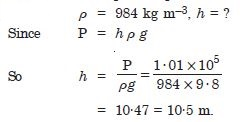##### Question 21:

A vertical off-shore structure is built to withstand a maximum stress of 109 Pa. Is the structure suitable for putting up of top of an oil well in the ocean ? Take the depth of ocean to be roughly 3 km, and ignore ocean currents.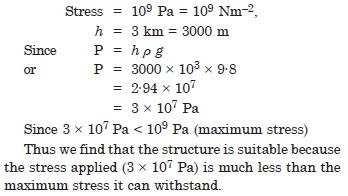##### Question 22:

A hydraulic automobile lift is designed to lift cars with a maximum mass of 3000 kg. The area of cross-section of the piston carrying the load is 425 cm2. What maximum pressure would the smaller piston have to bear ?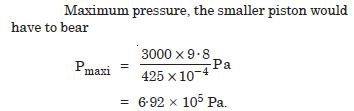##### Question 23:

A U-tube contains water and methylated spirit separated by mercury. The mercury columns in the two arms are in level with 10.0 cm of water in one arm and 12.5 cm of spirit in the other. What is the relative density of spirit ?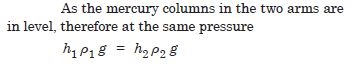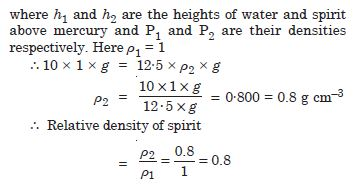##### Question 24:

In the previous problem, if 15·0 cm of water and spirit each are further poured into the respective arms of the tube, what is the difference in the levels of mercury, in the two arms ? (Relative density of mercury = 13.6).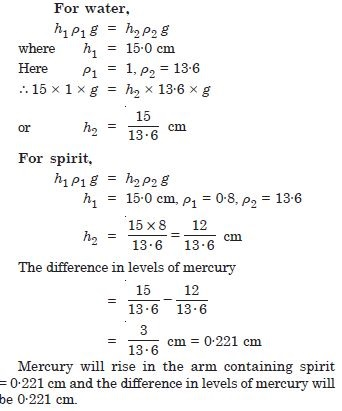##### Question 25:

Can Bernoulli’s equation be used to describe the flow of water through a rapid river ? Explain.

No, Bernoulli’s equation applies to streamline flow only and in a rapid river, the flow of water is not streamline.

##### Question 26:

Does it matter if one uses gauge instead of absolute pressure in applying Bernoulli’s equation ? Explain.

No, unless the atmospheric pressure at the two points where Bernoulli’s equation is applied are significantly different.

##### Question 27:

Glycerine flows steadily through a horizontal tube of length 1·5 m and radius 1·0 cm. If the amount of glycerine of collected per second at one end is 4·0 × 10–3 kg s–1. What is the pressure difference between the two ends of the tube, (density of glycerine = 1·3 × 102 kg m–3 and viscosity of glycerine = 0·83 Ns m–2) ? [You may also like to check if the assumption of laminar flow in the tube is correct].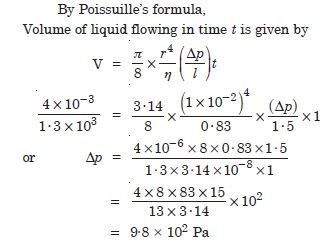##### Question 28:

In a test experiment on a model aeroplane in a wind tunnel, the flow speeds on the upper and lower surfaces of wings are 70 m s–1 and 63 m s–1 respectively. What is the lift on the wing if its area is 2·5 m2 ? Take the density of air to be 1·3 kg m3.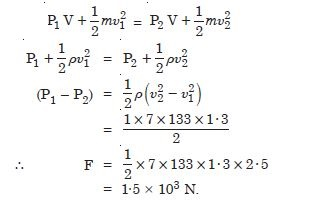##### Question 29:

Figures 10.2 (a) and (b) refer to the steady flow of a (non-viscous) liquid, which of the two figures is incorrect ? Why ?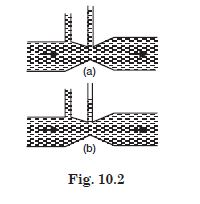Fig. (a) is incorrect. At a constriction (i.e. where the area of cross-section of the tube is smaller), flow speed is larger due to mass conservation. Consequently pressure there is smaller according to Bernoulli’s equation.

##### Question 30:

The cylindrical tube of a spray pump has a cross-section of 8·0 cm2 one end of which has 40 fine hole each of diameter 1·0 mm. If the liquid flows inside the tube 1.5 m per minute, what is the speed of ejection of liquid through the holes ?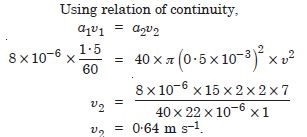##### Question 31:

A U-shaped wire is dipped in a soap solution and removed. The thin soap film formed between the wire and a light slider supports a weight of 1.5 × 10–2 N (which includes the small weight of the slider). The length of the slider is 30 cm. What is the surface tension of the film ?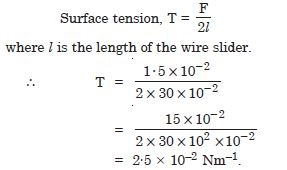##### Question 32:

Figure 10.3 (a) below shows a thin liquid film supporting a small weight = 4.5 × 102 N. What is the weight supported by a film of the same liquid at the same temperature in figures (b) and (c) ? Explain your answer physically.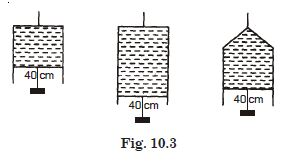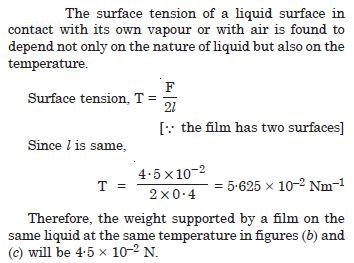##### Question 33:

What is the pressure inside a drop of mercury of radius 3·00 mm at room temperature ? Surface tension of mercury at that temperature (20$°$ C) is 4.65 × 10–1 Nm–1. The atmospheric pressure is 1·01 × 105 Pa. Also give the excess pressure inside the drop.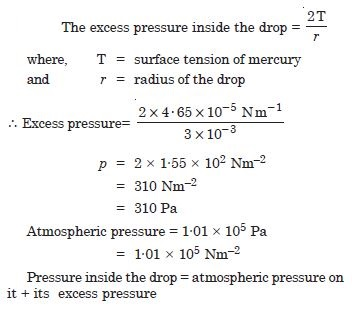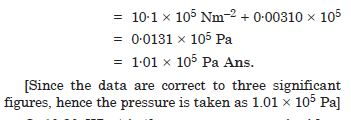What is the excess pressure inside a bubble of soap solution of radius 5.00 mm, given that the surface tension of soap solution at the temperature (20$°$ C) is 2.50 × 10–2 Nm–1 ? If an air bubble of the same dimension were formed at a depth of 40.0 cm inside a container containing the soap solution (of relative density 1.20), what would be the pressure inside the bubble ? (1 atm = 1.01 × 105 Pa).